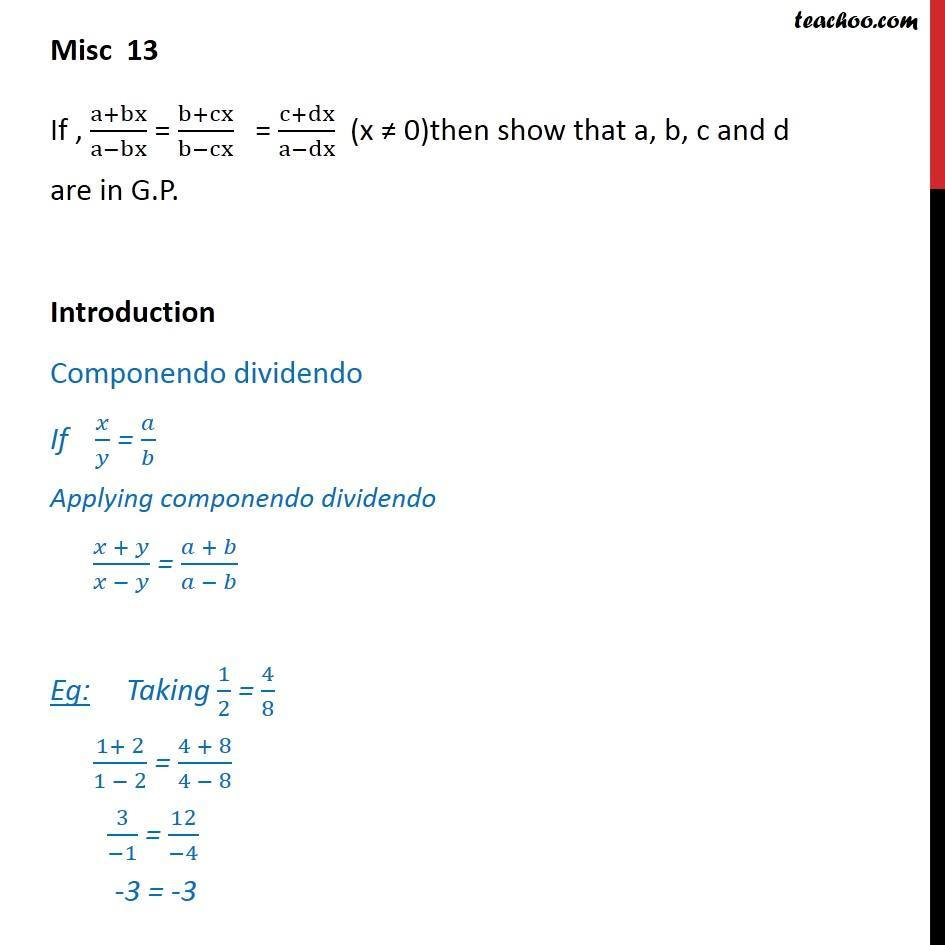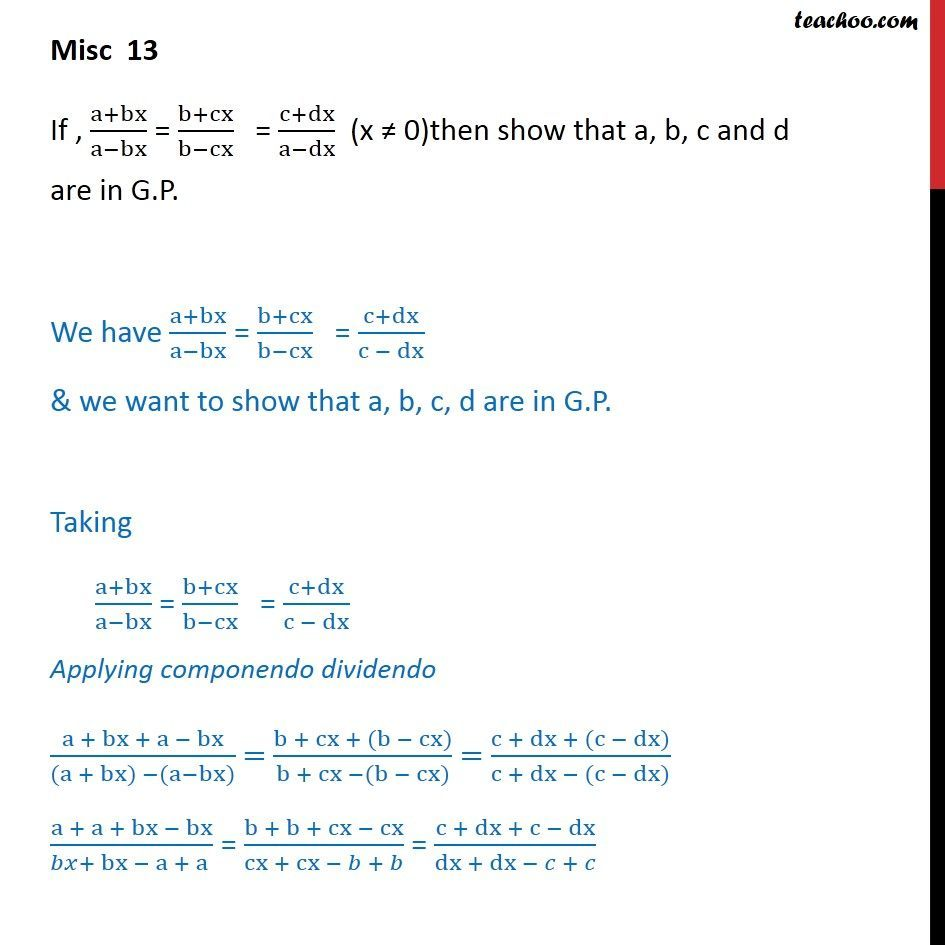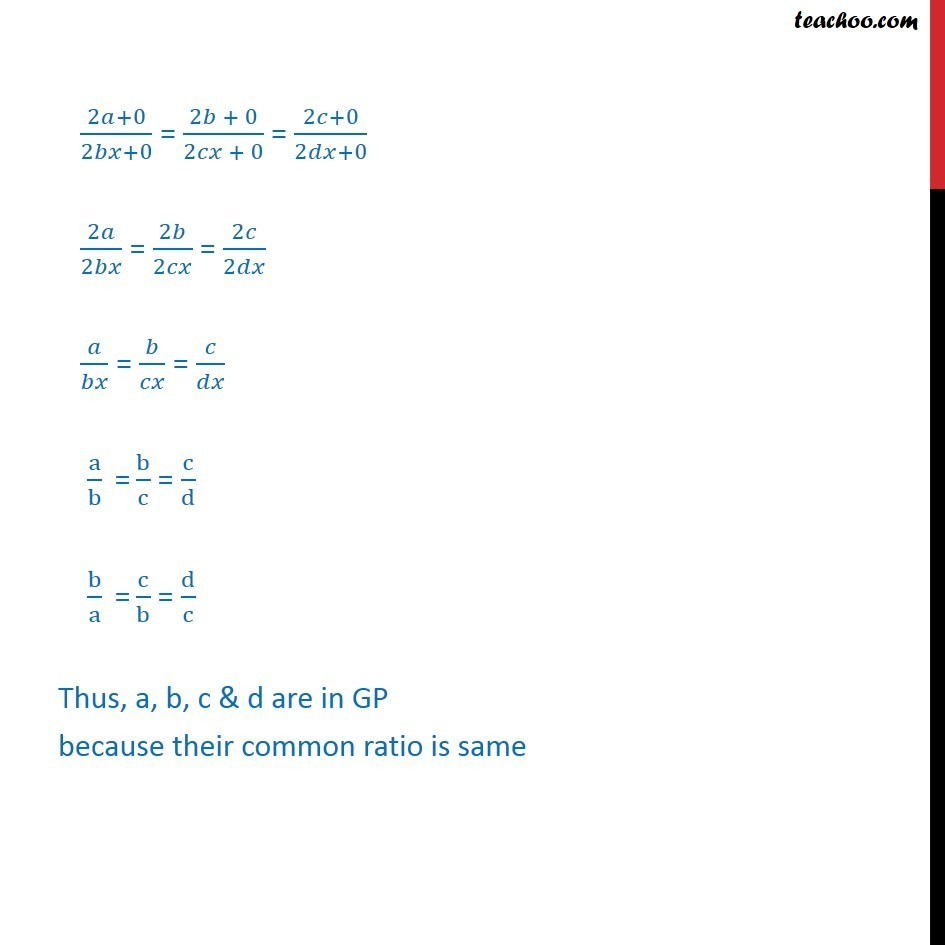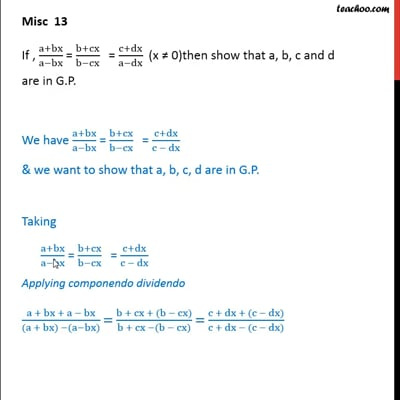Miscellaneous

Chapter 9 Class 11 Sequences and Series
Serial order wiseThis video is only available for Teachoo black users

Get live Maths 1-on-1 Classs - Class 6 to 12

### Transcript

Misc 13 If , (a+bx)/(a−bx) = (b+cx)/(b−cx) = (c+dx)/(a−dx) (x ≠ 0)then show that a, b, c and d are in G.P. Introduction Componendo dividendo If 𝑥/𝑦 = 𝑎/𝑏 Applying componendo dividendo (𝑥 + 𝑦)/(𝑥 − 𝑦) = (𝑎 + 𝑏)/(𝑎 − 𝑏) Eg: Taking 1/2 = 4/8 (1+ 2)/(1 − 2) = (4 + 8)/(4 − 8) 3/(−1) = 12/(−4) -3 = -3 Misc 13 If , (a+bx)/(a−bx) = (b+cx)/(b−cx) = (c+dx)/(a−dx) (x ≠ 0)then show that a, b, c and d are in G.P. We have (a+bx)/(a−bx) = (b+cx)/(b−cx) = (c+dx)/(c − dx) & we want to show that a, b, c, d are in G.P. Taking (a+bx)/(a−bx) = (b+cx)/(b−cx) = (c+dx)/(c − dx) Applying componendo dividendo (a + bx + a − bx)/((a + bx) −(a−bx)) = (b + cx + (b − cx))/(b + cx −(b − cx)) = (c + dx + (c − dx))/(c + dx − (c − dx)) (a + a + bx − bx)/(𝑏𝑥+ bx − a + a ) = (b + b + cx − cx)/(cx + cx − 𝑏 + 𝑏) = (c + dx + c − dx)/(dx + dx − 𝑐 + 𝑐) (2𝑎+0)/(2𝑏𝑥+0) = (2𝑏 + 0)/(2𝑐𝑥 + 0) = (2𝑐+0)/(2𝑑𝑥+0) 2𝑎/2𝑏𝑥 = 2𝑏/2𝑐𝑥 = 2𝑐/2𝑑𝑥 𝑎/𝑏𝑥 = 𝑏/𝑐𝑥 = 𝑐/𝑑𝑥 a/b " =" b/c = c/d b/a " =" c/b = d/c Thus, a, b, c & d are in GP because their common ratio is same